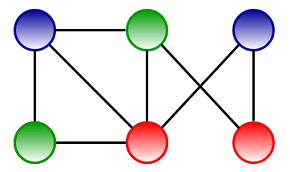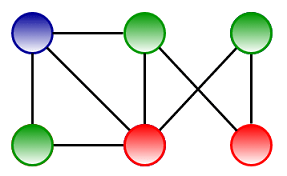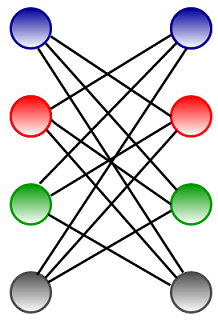# Greedy coloring of graph

The graph coloring (also called as vertex coloring) is a way of coloring the vertices of a graph such that no two adjacent vertices share the same color. In this post we will discuss a greedy algorithm for graph coloring and try to minimize the number of colors used.

For example, consider below graph,It can be colored in many ways by using minimum 3 colors.Please note that we can’t color the above graph using 2 colors.

Before discussing greedy algorithm to color graphs, lets talk about basic graph coloring terminology.

k-colorable graph –

A coloring using at most k colors is called a (proper) k-coloring and graph that can be assigned a (proper) k-coloring is k-colorable.

k-chromatic graph –

The smallest number of colors needed to color a graph G is called its chromatic number and a graph that is k-chromatic if its chromatic number is exactly k.

Brooks’ theorem –

It states that a connected graph can be colored with only x colors where x is maximum degree of any vertex in the graph except for complete graphs and graphs containing odd length cycle, which requires x + 1 colors.

Greedy coloring considers the vertices of the graph in sequence and assigns each vertex its first available color. i.e. vertices are considered in a specific order v1, v2, .. vn and vi is assigned the smallest available color which is not used by any of vi‘s neighbors.

Greedy coloring don’t always use the minimum number of colors possible to color a graph. For a graph of maximum degree x, greedy coloring will use at most x + 1 colors. Greedy coloring can be arbitrarily bad; for example, below crown graph (a complete bipartite graph) having n vertices can be 2-colored (refer left image), but greedy coloring resulted in n/2 colors (refer right image).## C++

Output:

Color assigned to vertex 0 is BLUE
Color assigned to vertex 1 is GREEN
Color assigned to vertex 2 is BLUE
Color assigned to vertex 3 is RED
Color assigned to vertex 4 is BLUE
Color assigned to vertex 5 is GREEN

## Java

Output:

Color assigned to vertex 0 is BLUE
Color assigned to vertex 1 is GREEN
Color assigned to vertex 2 is BLUE
Color assigned to vertex 3 is RED
Color assigned to vertex 4 is RED
Color assigned to vertex 5 is GREEN

#### Applications of graph coloring –

The problem of coloring a graph arises in many practical areas such as pattern matching, designing seating plans, scheduling exam time table, solving Sudoku puzzles, etc.

References:(1 votes, average: 5.00 out of 5)Loading...